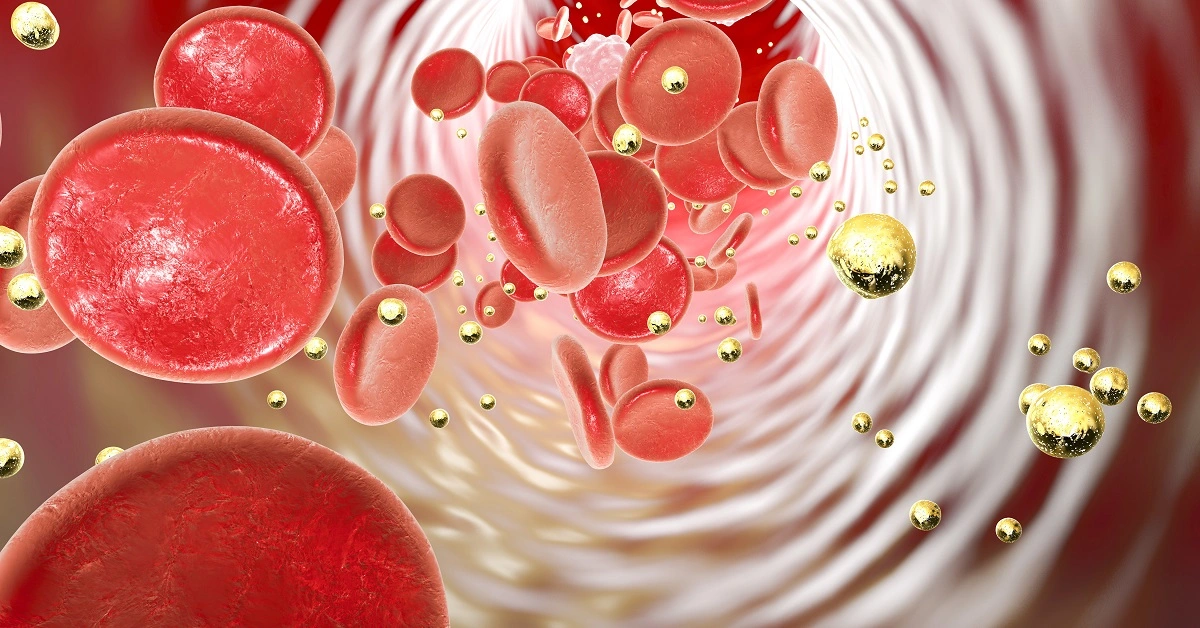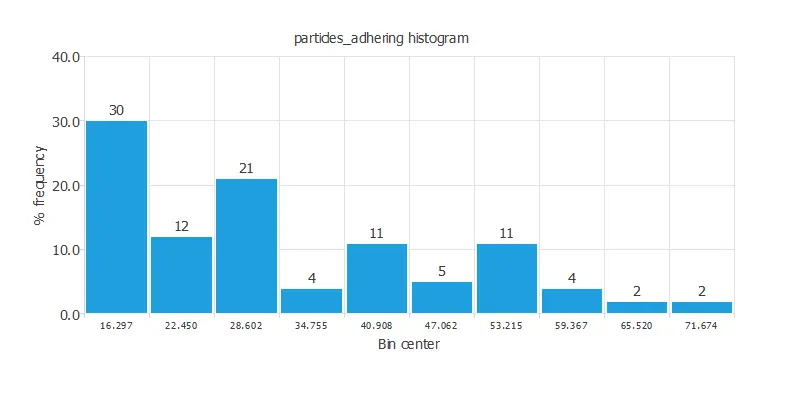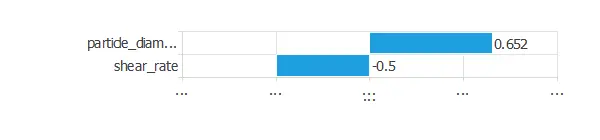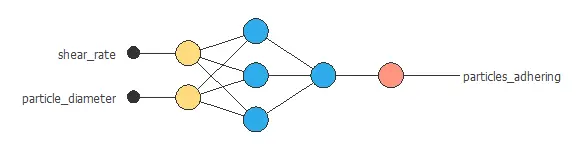# Model the adhesive strength of nanoparticles using Artificial Intelligence

The use of nanoparticles for the early diagnosis, treatment, and imaging of several disorders has increased recently.

Indeed, they can be administered at the systemic level transported by blood flow and reach any site within the macrovascular and microvascular circulation.

This study aims to predict the vascular adhesion of nanoparticles from their wall shear rate and diameter.### Contents:

This example is solved with Neural Designer. To follow it step by step, you can use the free trial.

## 1. Application type

This is an approximation project since the variable to be predicted is continuous (adhesive strength).

Here, the primary goal is to model the adhesive strength as a function of the shear rate and the particle diameter.

## 2. Data set

The data set contains three concepts:

• Data source.
• Variables.
• Instances.

The file nanoparticle_adhesive_strength.csv contains 58 rows, each of them with 3 columns.

This data set has the following variables:

• shear_rate: Wall shear rate of the nanoparticle. In 1/seconds, used as Input.
• particle_diameter: Diameter of each particle. In micrometers, used as Input.
• particles_adhering: Number of particles adhering per unit area to the collagen substrate. Used as Target.

For the analysis, we split the instances as follows: 60% for training and 40% for testing.

We can calculate the distributions of the variables. The following chart shows the histogram for the adhesion variable.As we can see, the adhesion has a semi-normal distribution.

The inputs-targets correlations help us to understand which input variables might have a bigger influence on the target variable.The particle diameter has a more significant correlation with the particles adhering than the shear rate. On the other hand, the particle diameter correlation is positive, and the shear rate correlation is negative.

## 3. Neural network

The second step is to set the model parameters. For approximation project type it is composed of:

• Scaling layer.
• Perceptron layers.
• Unscaling layer.

The mean and standard deviation is set as the scaling method, while the minimum and maximum is set as the unscaling method. The activation function chosen for this model is the hyperbolic tangent activation function and the linear activation function for the hidden layer and the output layer, respectively.

A graphical representation of the neural network is depicted next. It contains a scaling layer, two perceptron layers, and an unscaling layer. The number of inputs is 2, and the number of outputs is 1. The complexity, represented by the number of neurons in the hidden layer, is 3.## 4. Training strategy

The fourth step is to select an appropriate training strategy. It is composed of two things:

• A loss index.
• An optimization algorithm.

The loss index defines what the neural network will learn. It is composed of an error term and a regularization term.

The normalized squared error is used here in the error term. The regularization term is the L2 regularization. We use a weak weight for this regularization term.

The optimization algorithm is in charge of searching for the neural network parameters that minimize the loss index. Here we chose the quasi-Newton method as optimization algorithm.

The following chart shows how the error decreases with the iterations during the training process. The final values are training error = 0.062 NSE and selection error = 0.098 NSE, respectively.## 5. Model selection

The objective of model selection is to improve the generalization capabilities of the neural network or, in other words, to reduce the selection error.

Since the selection error that we have achieved so far is very small (0.098 NSE), we don't need to apply Order selection nor input selection here.

## 6. Testing analysis

Once we have trained the model, it is time to test its predictive capacity. This will be done by comparing the neural network outputs against the actual target values for a previously unseen data set. The testing analysis will determine if the model is ready to move to the production phase.

The next chart illustrates the linear regression analysis for the variable particles_adhering.For a perfect fit, the intercept, slope, and correlation values should be 0, 1, and 1. In this case, we have intercept = -2.57, slope = 1.11 and correlation = 0.946. The achieved values are close to the ideal ones, so the model performs well.

## 7. Model deployment

In the model deployment phase, the neural network is used to make predictions about the number of particles adhering to new values of wall share rate and diameter.

For that purpose, we can use Response Optimization. The objective of the response optimization algorithm is to exploit the mathematical model to look for optimal operating conditions.
Indeed, the predictive model allows us to simulate different operating scenarios and adjust the control variables to improve efficiency.

An example is to maximize particle diameter for a specific shear rate while maintaining the number of adhering particles over the desired value.

The next table resumes the conditions for this problem.

Variable name Condition
Shear rate Equal to 50
Particle diameter Maximize
Particle adhering Greater than or equal to 30

The next list shows the optimum values for previous conditions.

• shear_rate: 50/second.
• particle_diameter: 6.59259 micrometers.

We can plot Directional outputs to study the behavior of the output variable particle_adhering as the function of single inputs. The following reference point is used:

• shear_rate: 73.421 1/seconds.
• particle_diameter: 3.388 micrometers.

The following picture shows the number of particles adhering as a function of the wall shear rate around the reference point.As we can see, for this value of the particle diameter, the number of particles adhering keeps more or less constant until the wall shear rate reaches the values around 70, it starts decreasing.

On the other hand, the following image represents the number of particles adhering as a function of the particle diameter and a reference point of the wall shear rate with a value of 73.4211.In this case, for this value of the wall shear rate, the number of particles adhering increases until the particle diameter reaches the value 5, it starts decreasing.

As well, we can use the mathematical expression of the neural network, which is listed next.

```scaled_shear_rate = 2*(shear_rate-50)/(90-50)-1;
scaled_particle_diameter = (particle_diameter-3.38896)/2.38932;

y_1_1 = tanh(0.767924+ (scaled_shear_rate*-0.811769)+ (scaled_particle_diameter*2.21102));
y_1_2 = tanh(2.08552+ (scaled_shear_rate*-1.88506)+ (scaled_particle_diameter*-1.04971));